Wir verwenden Google für unsere Suche. Mit Klick auf „Suche aktivieren“ aktivieren Sie das Suchfeld und akzeptieren die Nutzungsbedingungen.

# New in SG-Lib 3.6

###### SGofCPLzdelaunayGrid(CPL,z,ds,dx,dy)- returns a solid from a contour polygon list and grid resolution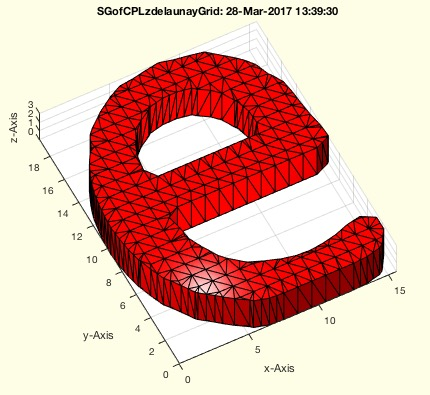% SGofCPLzdelaunayGrid(CPL,z,ds,dx,dy) - returns a solid from a contour polygon list and grid resolution% (by Tim Lüth & Christina Hein, VLFL-Lib, 2017-MÄR-27 as class: MODELING % PROCEDURES)% % The fnctn uses Delaunay-Triagulation to tesselate the base plate and % the cover plate of the part. Therefor, make sure that there are no % crossings or overlapping contours in CPL.% This fnctn will replace SGofCPLz one day since it has the same result % for the same parameter (Status of: 2017-03-29)% % See also: PLFLofCPLdelaunayGrid, CPLaddauxpoints% % SG=SGofCPLzdelaunayGrid(CPL,z,[ds,dx,dy])% === INPUT PARAMETERS ===% CPL: Contour polygon list (CPL)% z: desired height in z-coordiantes% ds: delta on the contour; default is 10000% dx: delta grid in x; default is 10000% dy: delta grid in y; default is dx% === OUTPUT RESULTS ======% SG: Solid Geometry% % EXAMPLE: SGofCPLzdelaunayGrid(CPLofPL([PLcircle(20,6);NaN NaN; % flipud(PLcircle(10,4))]),10)% SGofCPLzdelaunayGrid(CPLoftext('test'),10,4,4,4)%
###### CPLaddauxpoints(CPL,d)- add supporting points to embedded CPL to guarantee a specified points distance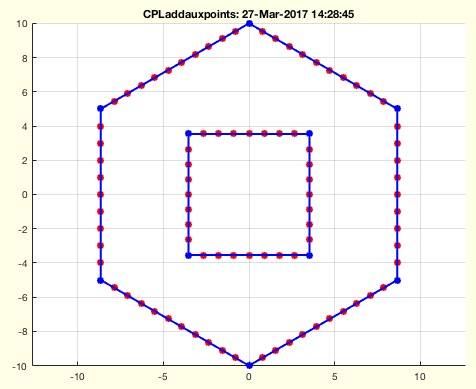% CPLaddauxpoints(CPL,d) - add supporting points to embedded CPL to guarantee a specified points distance% (by Tim Lüth & Christina Hein, VLFL-Lib, 2017-MÄR-27 as class: % AUXILIARY PROCEDURES)% % See also: SGofCPLzdelaunayGrid, PLFLofCPLdelaunayGrid, RLdelauxpoints, % GPLauxgridpointsCL, PLELaddauxpoints, GPLauxgridpointsPLEL, % RLaddauxpoints% % CPLN=CPLaddauxpoints(CPL,d)% === INPUT PARAMETERS ===% CPL: Closed Polygon List% d: maximum distance between two points% === OUTPUT RESULTS ======% CPLN: New Closed Polygon List% % EXAMPLE: % CPLaddauxpoints([PLcircle(10,6);NaN NaN; PLcircle(5,4)],1)% CPLaddauxpoints(CPLsample(12),1)%
###### PLFLofCPLdelaunayGrid(CPL,ds,dx,dy)- returns a point list and facet list for a grid filled contour list% PLFLofCPLdelaunayGrid(CPL,ds,dx,dy) - returns a point list and facet list for a grid filled contour list% (by Tim Lüth & Christina Hein, VLFL-Lib, 2017-MÄR-27 as class: SURFACES)% % See also: SGofCPLzdelaunayGrid, CPLaddauxpoints% % [PL,FL,EL]=PLFLofCPLdelaunayGrid(CPL,[ds,dx,dy])% === INPUT PARAMETERS ===% CPL: Closed Polygon list% ds: delta on contour; default is 4% dx: delta in x; default is ds% dy: delta in y; default is dx% === OUTPUT RESULTS ======% PL: Resulting Point list% FL: Resulting Facet list% EL: Resulting Edge List% % EXAMPLE: % PLFLofCPLdelaunayGrid ([PLcircle(20,6);NaN NaN;PLcircle(8,7)]);% CPL=CPLofPL([PLcircle(20);NaN NaN; flipud(PLcircle(10))]);% PLFLofCPLdelaunayGrid(CPL,2,2)% PLFLofCPLdelaunayGrid (PLcircle(20,6))%
###### VMgetSubplot- returns the current active subplot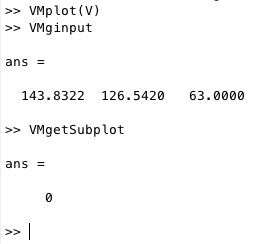% VMgetSubplot - returns the current active subplot% (by Tim Lueth, VLFL-Lib, 2017-MÄR-21 as class: USER INTERFACE)% % This fnctn returns for the VMplot figure, the number of the subplot, of % the last VMginput position was taken (Status of: 2017-03-21)% % See also: VMginput, VMplot% % subpn=VMgetSubplot% === OUTPUT RESULTS ======% subpn: current subplot position; empty if figure is not valid%
###### getCurrentPoint- this function returns the currentPoint but also the axis limits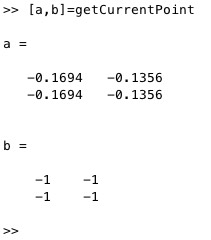% getCurrentPoint - this fnctn returns the currentPoint but also the axis limits% (by Tim Lueth, VLFL-Lib, 2017-MÄR-21 as class: USER INTERFACE)% % Sometime after ginput or after get(gca,'CurrentPoint' ) the returned % coordinates are unfortunately not inside of the active axis. This % Matlab bug is related to changing programmatically the size of the % window by a command and having at the time the windowchange allowed.% This fnctn just help to detect the problem. So it is more a debug % fnctn. (Status of: 2017-03-27)% % See also: view, get(gca, 'CurrentPoint', )% % [p,ax]=getCurrentPoint% === OUTPUT RESULTS ======% p: 2 point describing the view axis of the last click% ax: axis limits for each point -1 to small + 1 to big 0=inside of view%
###### VMginput(n,u)- returns a point position by clicking into VMplot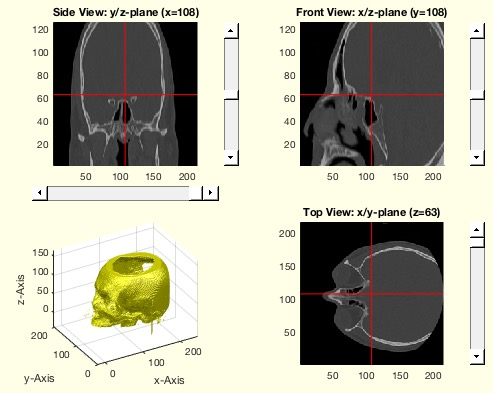% VMginput(n,u) - returns a point position by clicking into VMplot% (by Tim Lueth, VLFL-Lib, 2017-MÄR-20 as class: USER INTERFACE)% % This fnctn is based on ginput, waitforbuttonpress, % get(gca,'CurrentPoint').% If the window is magnified, it has to be in the center of the screen % (Status of: 2017-03-21)% % See also: VMplot, VMgetSubplot% % [PL,t]=VMginput([n,u])% === INPUT PARAMETERS ===% n: number of points% u: update VMplot; default is true% === OUTPUT RESULTS ======% PL: position% t: subplot%
###### SGtitle(n,w)- draws the name of the calling function as figure title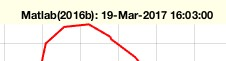% SGtitle(n,w) - draws the name of the calling fnctn as figure title% (by Tim Lueth, Video-Lib, 2017-MÄR-19 as class: AUXILIARY PROCEDURES)% % same as title(titleofcaller,'Interpreter','none');% (Status of: 2017-03-19)% % See also: titleofcaller% % SGtitle([n,w])% === INPUT PARAMETERS ===% n: hierarchy of calling fnctns; default is 0;% w: 'window' renames the window instead of a gca title% % EXAMPLE: % SGfigure; SGtitle% SGfigure; SGtitle(1)% SGfigure; SGtitle(-1)%
###### exp_2017_03_18a(A,comp)- EXPERIMENT to calculate bended surfaces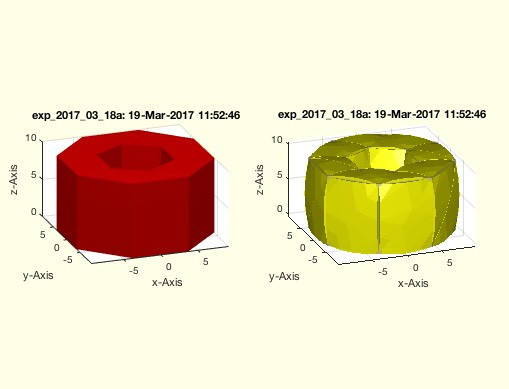% exp_2017_03_18a(A,comp) - EXPERIMENT to calculate bended surfaces% (by Tim Lueth, VLFL-Lib, 2017-MÄR-19 as class: EXPERIMENTS)% % By compressing a solid, the edges are compressed but the length of the % original edges have to be constant. The bending curve is delivered by % rofcircbend. This is done for each facet edge. In this example in % addition also the resulting solid for each face is calculated and shown.% IN THIS EXPERIMENT EACH FACET is handled separately. For complete % solids it is necessary to handle edges instead of faces. Each edge will % result in one ore more (grow) contours. (Status of: 2017-03-19)% % exp_2017_03_18a([A,comp])% === INPUT PARAMETERS ===% A: Solid Geometry% comp: compression such as 0.95 or 0.9%
###### rofcircbend(s,l)- returns bending radius and angle for a compressed line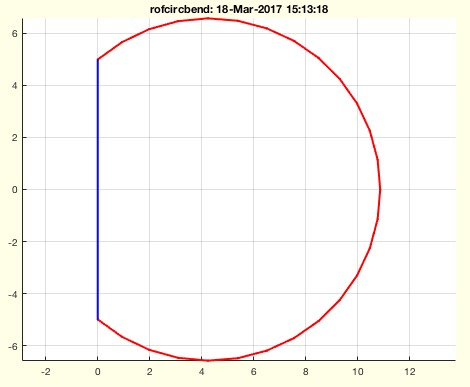% rofcircbend(s,l) - returns bending radius and angle for a compressed line% (by Tim Lueth, VLFL-Lib, 2017-MÄR-18 as class: ANALYTICAL GEOMETRY)% % the relation between a circle segment and the chord (segment crossing % line) can be solved only by a numerical search using fzero.% if s < l than the line is streched.% if s = l than the radius would be inf% if s > l there is a solution% In reality there is a minimal radius for bending because of material % stress (Status of: 2017-03-19)% % See also: lofbendinggirder, sofbendinggirder% % [r,a,PL]=rofcircbend(s,l)% === INPUT PARAMETERS ===% s: original distance% l: compressed distance% === OUTPUT RESULTS ======% r: bending radius% a: bending angle% PL: Point list describing the shape% % EXAMPLE: % rofcircbend(30,10)%
###### exp_2017_03_18- EXPERIMENT to solve transcendent functioon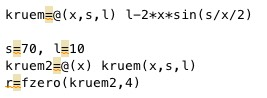% exp_2017_03_18 - EXPERIMENT to solve transcendent functioon% (by Tim Lueth, VLFL-Lib, 2017-MÄR-18 as class: EXPERIMENTS)% % l=2*r*sin(alpha) = 2*r*sin(b/(2*r)% alpha=b/r% (Status of: 2017-03-18)% % exp_2017_03_18%
###### CVLofcontourc(C,rem)- converts the contourc format into the CVL format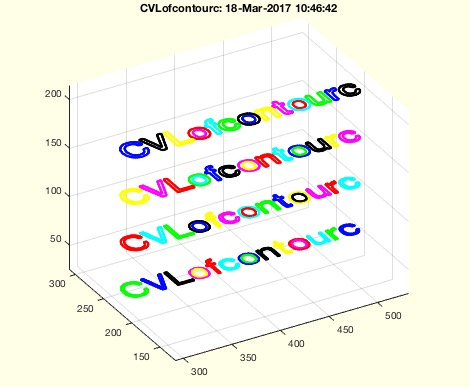% CVLofcontourc(C,rem) - converts the contourc format into the CVL format% (by Tim Lueth, VLFL-Lib, 2017-MÄR-18 as class: CLOSED POLYGON LISTS)% % Matlab's contourc fnctn is a powerful fnctn to create contours from % image bit maps similar to the marching cube fnctn in 3D. Nevertheless % the resulting point list format is outdated. This fnctn converts the % contourc format into the CVL format. The z values are the contourc % intesity(z) values. (Status of: 2017-03-18)% % See also: CPLofcontourc, contourc, CPLofimage, SGofVMdelaunay, % SGofVMmarchcube, CPLremstraight% % CVL=CVLofcontourc(C,[rem])% === INPUT PARAMETERS ===% C: result of the contourc fnctn% rem: removes straight lines if true; default is false% === OUTPUT RESULTS ======% CVL: Closed polygon list [nx3] with the z values for the contour% % EXAMPLE: % figure; axis off; text(0.5,0.5,'This is a Test', 'FontSize',36)% I=getframe(gcf); % CVLofcontourc(contourc(1/3*sum(flipud(I.cdata),3),4))%
###### CPLcontourc(Inputparameter)- returns the CPL of matlab's contourc command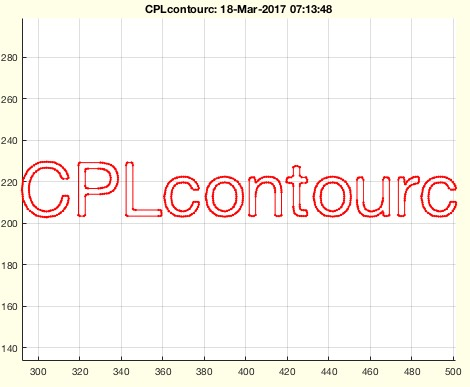% CPLcontourc(Inputparameter) - returns the CPL of matlab's contourc command% (by Tim Lueth, VLFL-Lib, 2017-MÄR-18 as class: CLOSED POLYGON LISTS)% % This fnctn simply calls contourc and converts the output matrix into % CPL format.% For the properties of the contour matrix result read the help for % ContourMatrix% ATTENTION: The original ContourMatrix supports open and closed PL! % ATTENTION: The original ContourMatrix has many points on a staight line!% Automatically converts the type into the requested double format. % (Status of: 2017-05-24)% % See also: PLofimcontourc, CVLofcontourc, CPLofcontourc, contourc, % ContourMatrix, % https://de.mathworks.com/help/matlab/ref/contour-properties.html% % CPL=CPLcontourc([Inputparameter])% === INPUT PARAMETERS ===% Inputparameter: exactly the parameter same as contourc% === OUTPUT RESULTS ======% CPL: Closed polygon list % % EXAMPLE: % figure; axis off; text(0.5,0.5,'This is a Test', 'FontSize',36)% I=getframe(gcf); I=flipud(I.cdata(:,:,1)); % CPLcontourc(I,1); %
###### smbCreateSpring(M1,M2,SGName,len,sprg,damp)- smb creates a spring damper link between two point masses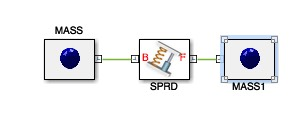% smbCreateSpring(M1,M2,SGName,len,sprg,damp) - smb creates a spring damper link between two point masses% (by Tim Lueth, SimMechanics, 2017-MÄR-17)% % M1 and M2 are connected using LConn1 (Status of: 2017-03-17)% % See also: smbNewSystem, smbCreateSGMass% % h=smbCreateSpring(M1,M2,[SGName,len,sprg,damp])% === INPUT PARAMETERS ===% M1: Mass 1% M2: Mass 2% SGName: optional name; default is 'SPRD;% len: len in mm% sprg: spring constant in N/m% damp: Damping in N/(m/s)% === OUTPUT RESULTS ======% h: spring-damper block% % EXAMPLE: % smbNewSystem ('smb_exp_2017_03_17b',[0 0 -9.81]);% M1=smbCreateSGMass;% M2=smbCreateSGMass;% smbCreateSpring(M1,M2);% smbAddLine( 'WORLD/RConn1', [M1 '/LConn1']);%
###### smbCreateSGMass (SG,SGName,SGcol)- smbcreates a double mass model with random inertia and point mass% smbCreateSGMass (SG,SGName,SGcol) - smbcreates a double mass model with random inertia and point mass% (by Tim Lueth, SimMechanics, 2017-MÄR-17 as class: MODELING PROCEDURES)% % Since smb start the movement in the origin, it is very easy to create % singularity condition without intention. Therefor, this fnctn links a % point mass with a random inertia deviation to create objects that can % be connected by springs (Status of: 2017-03-17)% % See also: smbNewSystem, smbCreateSpring% % smbCreateSGMass([SG,SGName,SGcol])% === INPUT PARAMETERS ===% SG: Solid Geometry; default is a sphere with radius 1% SGName: Optional Name; default is 'MASS'% SGcol: Optional Color% % EXAMPLE: % smbNewSystem ('smb_exp_2017_03_17b',[0 0 -9.81]);% M1=smbCreateSGMass;% M2=smbCreateSGMass;% smbCreateSpring(M1,M2);% smbAddLine( 'WORLD/RConn1', [M1 '/LConn1']);%
###### exp_2017_03_17- EXPERIMENT to show falling and oscillation of a mass point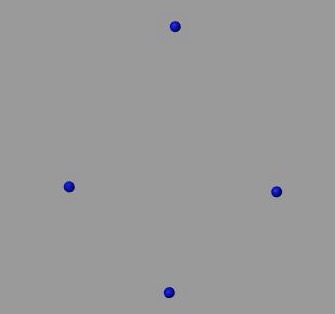% exp_2017_03_17 - EXPERIMENT to show falling and oscillation of a mass point% (by Tim Lueth, VLFL-Lib, 2017-MÄR-17 as class: EXPERIMENTS)% % This is the basic experiment for mass spring damper systems, starting % the simulation all in the origin (Status of: 2017-03-17)% % exp_2017_03_17%
###### SGmodelMass(R)- creates a mass point including 2 frames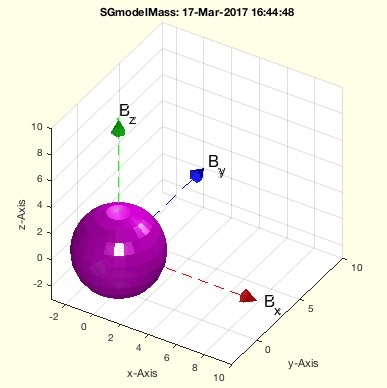% SGmodelMass(R) - creates a mass point including 2 frames% (by Tim Lueth, VLFL-Lib, 2017-MÄR-17 as class: SIMMECHANICS INTERFACE)% % See also: SGmodelLink, SGmodelJoint, SGmodelNode, SGmodelLink3, % SGmodelKeyhole, SGmodelLink1, SGmodelLink2% % SG=SGmodelMass([R])% === INPUT PARAMETERS ===% R: Radius% === OUTPUT RESULTS ======% SG: Solid geometry% % EXAMPLE: % SGmodelMass(8)%
###### CPLoftext(str,siz,FW,FN)- returns a CPL of one or more textlines separated by \n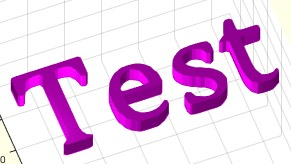% CPLoftext(str,siz,FW,FN) - returns a CPL of one or more textlines separated by \n% (by Tim Lueth, VLFL-Lib, 2017-MÄR-17 as class: CLOSED POLYGON LISTS)% % This fnctn takes seconds to process.% 10 point ~ 7 mm "g' to 'X' is about 10mm, a line is about 14 mm (Status % of: 2017-03-17)% % See also: CPLofimage, CPLofcontourc, textHorizontalBlockAlign% % CPL=CPLoftext(str,[siz,FW,FN])% === INPUT PARAMETERS ===% str: one or more textlines separated by \n% siz: size in pt% FW: FontWidth; see text; default is 'normal'% FN: FontName; see text; default is 'Helevetica'% === OUTPUT RESULTS ======% CPL: Closed Polygon Line% % EXAMPLE: % tic; CPLoftext('test',0.2); toc;% tic; CPLoftext('test',70); toc;% SGofCPLz(CPLoftext('test'),5); view(-30,30);% CPLoftext('The quick brown fox jumps\n over the lazy % dog',10,'bold','Cambria')%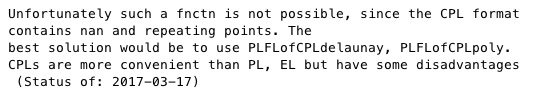% FLofCPL(CPL) - misleading fnctn - use PLFLofCPLdelaunay, PLFLofCPLpoly instead% (by Tim Lueth, Video-Lib, 2017-MÄR-17 as class: CLOSED POLYGON LISTS)% % Unfortunately such a fnctn is not possible, since the CPL format % contains nan and repeating points. The best solution would be to use % PLFLofCPLdelaunay or PLFLofCPLpoly.% CPLs are more convenient than PL, EL but have some disadvantages% (Status of: 2017-03-17)% % See also: PLFLofCPLpoly, PLFLofCPLdelaunay, PLELofCPL, FLofPLEL% % FL=FLofCPL(CPL)% === INPUT PARAMETERS ===% CPL: Closed Polygon List% === OUTPUT RESULTS ======% FL: Facet List% % EXAMPLE: Try instead% PLFLofCPLdelaunay(CPL)% %
###### CPLofcontourc(C,rem)- converts the contourc format into the CPL format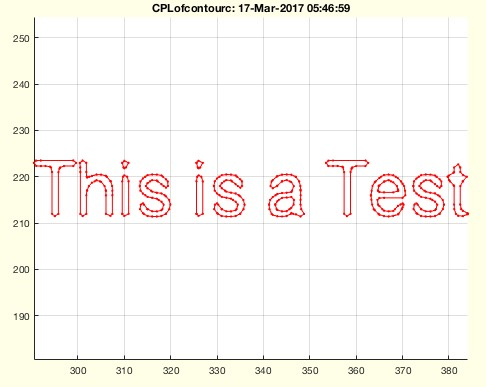% CPLofcontourc(C,rem) - converts the contourc format into the CPL format% (by Tim Lueth, VLFL-Lib, 2017-MÄR-17 as class: CLOSED POLYGON LISTS)% % Matlab's contourc fnctn is a powerful fnctn to create contours from % image bit maps similar to the marching cube fnctn in 3D. Nevertheless % the resulting point list format is outdated. This fnctn converts the % contourc format into the CPL format. (Status of: 2017-03-18)% % See also: CVLofcontourc, contourc, CPLofimage, SGofVMdelaunay, % SGofVMmarchcube, CPLremstraight% % CPL=CPLofcontourc(C,[rem])% === INPUT PARAMETERS ===% C: result of the contourc fnctn% rem: removes straight lines if true; default is true% === OUTPUT RESULTS ======% CPL: Closed polygon list% % EXAMPLE: % figure; axis off; text(0.5,0.5,'This is a Test', 'FontSize',36)% I=getframe(gcf); I=flipud(I.cdata(:,:,1)); C=contourc(double(I),1); % CPLofcontourc(C)%
###### VLofimage(I,pixsize,h,nf)- converts an image into a contour or a solid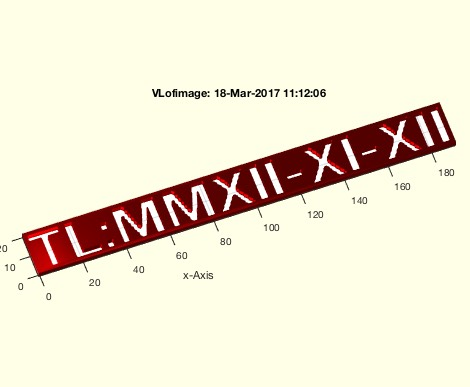% VLofimage(I,pixsize,h,nf) - converts an image into a contour or a solid% (by Tim Lueth, VLFL-Lib, 2017-MÄR-16 as class: TEXT PROCEDURES)% % Rewritten based on the contour fnctn. Quite useful procedure to % generate contours from images or string comments in combination with % imageoftext. If parameter h>0, the fnctn returns a solid.% There is an earlier version, now called VLofimage_2012b. (Status of: % 2017-03-18)% % See also: CPLtextimage, VLFLtextimage, imageoftext% % [VL,EL,FL,CPL]=VLofimage(I,[pixsize,h,nf])% === INPUT PARAMETERS ===% I: image array% pixsize: size of a pixel% h: desired heigth; default is 0 (Contour)% nf: frame in pixels; if nf>0 the image is impressed; default is 0% === OUTPUT RESULTS ======% VL: Vertex list (nx2) for h=0; (nx3) for h>0;% EL: Edge list% FL: Facet list% CPL: Closed Polygon list based on contour% % EXAMPLE: Generate a contour for the string 'Test':% I=imageoftext('TL:MMXII-XI-XII',36); % [VL,EL,FL]=VLofimage(I,.7); VLELplot(VLaddz(VL),EL,'r-')% [VL,EL,FL]=VLofimage(I,.7,3); close all, VLFLplot(VL,FL),%
###### CPLofimage(I,n,f)- returns a point list related to matlab;s contour function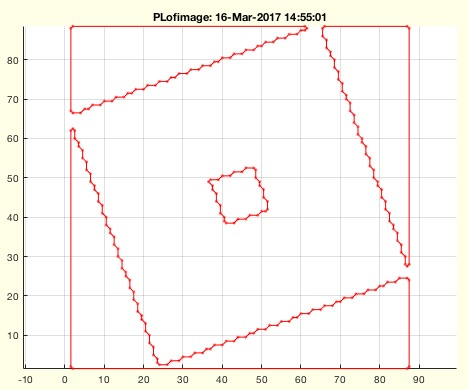% CPLofimage(I,n,f) - returns a point list related to matlab;s contour fnctn% (by Tim Lueth, VLFL-Lib, 2017-MÄR-16 as class: CLOSED POLYGON LISTS)% % based on matlab's contour to accelerate the fnctn VLofimage. If image I % is a struct, I is converted into a gray scale image by: % I=1/s(3)*sum(I.cdata,3)% (Status of: 2017-03-17)% % See also: CPLremstraight, VLofimage% % CPL=CPLofimage(I,[n,f])% === INPUT PARAMETERS ===% I: image% n: number of contours% f: if true create a zero frame around; default is false% === OUTPUT RESULTS ======% CPL: Closed Polygon List% % EXAMPLE: % figure; axis off; text(0.5,0.5,'This is a Test', 'FontSize',16); % I=getframe(gcf)% CPLofimage(I)% CPLofimage(I.cdata(:,:,1)<240)% I.cdata=squeeze(I.cdata(:,:,1)); CPLofimage(I)%
###### CPLsortinout(CPL,in1st)- returns a inside outside sorted CPL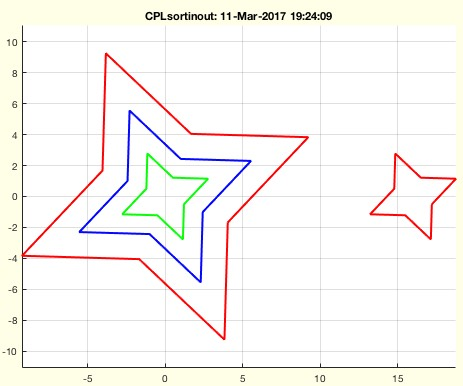% CPLsortinout(CPL,in1st) - returns a inside outside sorted CPL% (by Tim Lueth, VLFL-Lib, 2017-MÄR-11 as class: CLOSED POLYGON LISTS)% % For laser cutting, the insider first order is required. For automatic % detection of corresponding vessels, outside first is important.% Corresponance matrix: % (i,j)==+1 i is inside j, -1== i is outside j, NaN==crossing% (Status of: 2017-06-04)% % See also: connectofmat, selectNaN, separateNaN, CPLisccwinout, % svgpolylineofCPL, CPLwriteSVG% % [cind,CC,NCPL,pi]=CPLsortinout([CPL,in1st])% === INPUT PARAMETERS ===% CPL: Original CPL% in1st: true==inside first; false=outside first; default is true% === OUTPUT RESULTS ======% cind: level index related to separatedNaN (CPL)% CC: Corresponance matrix% NCPL: New contour list sorted by enclosure order% pi: parent index list% % EXAMPLE: % CPLsortinout(selectNaN(CPLsample(14),[1,2,3,6]))% CPLsortinout(selectNaN(CPLsample(14),[1,2,3,6]),false)% [~,CC]=CPLsortinout(CPLsample(14)); c=connectofmat(CC)%
###### selectNaN(CPL,ci)- connects subsets of closed polygons lines (2D/3D) wrt an index list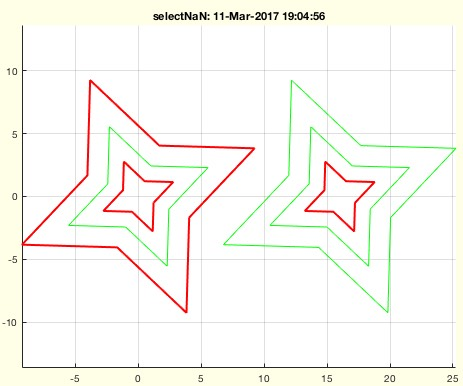% selectNaN(CPL,ci) - connects subsets of closed polygons lines (2D/3D) wrt an index list% (by Tim Lueth, VLFL-Lib, 2017-MÄR-11 as class: CLOSED POLYGON LISTS)% % See also: separateNaN, replaceNaN, cellofNaN% % CPLN=selectNaN(CPL,ci)% === INPUT PARAMETERS ===% CPL: CPL / CVL% ci: index list [% === OUTPUT RESULTS ======% CPLN: Subset of CPL or new ordered CPL% % EXAMPLE: Select 3 CPL of CPLsample(14)% selectNaN(VLaddz(CPLsample(14),pi),[1,3,6])%
###### movepathtotop (pstr)- moves a search path from its current position to the beginning of the search path% movepathtotop (pstr) - moves a search path from its current position to the beginning of the search path% (by Forian Schleich & Tim Lueth, VLFL-Lib, 2017-MÄR-11 as class: % AUXILIARY PROCEDURES)% % Some libraries such as VLFL-Lib or SG-Lib overload unfortunately % existing fnctns such as roundN. Nevertheless, by using the fnctn the % relevant library can be moved to the top. (Status of: 2017-03-11)% % See also: VLFLlicense% % movepathtotop([pstr])% === INPUT PARAMETERS ===% pstr: fullpath of a search directory; default is rec of %
###### RRcputime(RRtictime)- returns realtime difference and cputime difference since first call% RRcputime(RRtictime) - returns realtime difference and cputime difference since first call% (by Christian Dietz, VLFL-Lib, 2017-MÄR-09 as class: AUXILIARY % PROCEDURES)% % If Matlab works with 100% CPU time, tic time and CPU time are % identical. Nevertheless, is makes sense also to check the Realtime in % realtime systems :-) (Status of: 2017-03-09)% % See also: RRrun% % [t,tc]=RRcputime([RRtictime])% === INPUT PARAMETERS ===% RRtictime: only used for reset by tic% === OUTPUT RESULTS ======% t: time difference since first call% tc: CPUtime difference since first call%
###### plotannotation(str,)- plots a annotation box into the right upper corner of a plot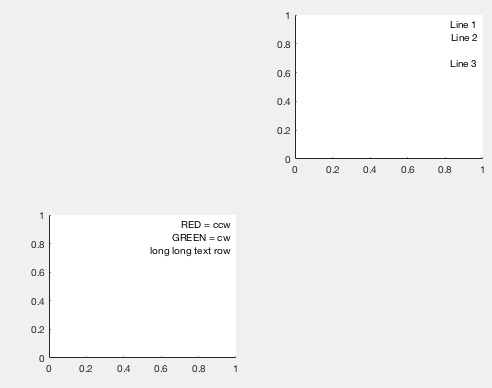% plotannotation(str,) - plots a annotation box into the right upper corner of a plot% (by Tim Lueth, VLFL-Lib, 2017-MÄR-09 as class: USER INTERFACE)% % the annotation box is able to adjust the size but this fnctn is related % to the "left" "HorizontalAlignment". Therefor it is necessary to % calculate a little to bring an automatical size adjusted box into the % right corner of a subplot (Status of: 2017-03-09)% % See also: annotation, titleofcaller% % t=plotannotation(str,[])% === INPUT PARAMETERS ===% str: string or cell with separated strings% === OUTPUT RESULTS ======% t: handle to annotation box% % EXAMPLE: % subplot(2,2,3); % t=plotannotation({'RED = ccw','GREEN = cw','long long text % row'},'linestyle','none'); % subplot(2,2,2);% t=plotannotation('Line 1\n Line 2\n\nLine 3','linestyle','none'); % %